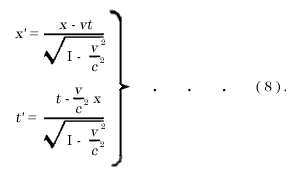# Thread: Mathematical equations and formulas

1. ##Originally Posted by shoshanatis a beautiful equation i hope it can not be disprovedhttp://www.nytimes.com/2011/09/30/op...as30.html?_r=1
Holy crap, they can't make the theory wrong because of 60 nanoseconds! Specially because Einstein is the most famous INTP representative.Reply With Quote

2. ##Originally Posted by _Poki_Soh Cah Toa

its the one I have found the most useful
Related: Cao Sha Cho.Originally Posted by gmanyoI've always hated equations. Memorizing boring shit that I don't care about. If I had to pick one I'd go with f'(x) = (limit as h approaches 0) f(x+h) - f(x) / h (the function for derivatives) because derivatives and integrals are awesome concepts
Another useful formula: f'(x)=y+dy=f(x+dx). Solve for dy/dx to find the derivative. Equally effective and much easier than limits.Originally Posted by gmanyo...and that equation is less about memorizing what numbers go where than it is about understanding larger ideas.
That's what math is about, and it often isn't taught that way.

Other than that, I tend to like miscellaneous mathematical formulae I've derived.Reply With Quote

3. ##Also recently just learned about Lorentz Transformations (from Yale!):Really awesome. Relates how people view time, space, order of events, length of objects, etc. from relative velocity. Go Einstein!
(These are the main equations of relativity which lead to the more famous E=mc^2)Reply With Quote

4. ##Originally Posted by SaslouHey guys ..

I would like to know what your all time favourite mathematical equation/formula is.
But I hate maths.Reply With Quote

5. ##Originally Posted by HeliosBut I hate maths.

That's OK.. Just rep me your all time favourite <Insert anything here>Reply With Quote

6. ##The Gaussian distribution:Reply With Quote

7. ##If I understood the Schrödinger equation I would probably like that one.Reply With Quote

8. ##Bayes' rule.

It's magical.Reply With Quote

9. ##WTF? People actually have favorite formulas? This is news to me.Reply With Quote

10. ##Ohm's Law
For many conductors of electricity, the electric current which will flow through them is directly proportional to the voltage applied to them. When a microscopic view of Ohm's law is taken, it is found to depend upon the fact that the drift velocity of charges through the material is proportional to the electric field in the conductor. The ratio of voltage to current is called the resistance, and if the ratio is constant over a wide range of voltages, the material is said to be an "ohmic" material. If the material can be characterized by such a resistance, then the current can be predicted from the relationship:

Ohm's Law - I=V/R or Electric Current = Voltage / ResistanceReply With Quote

#### Posting Permissions

• You may not post new threads
• You may not post replies
• You may not post attachments
• You may not edit your posts
Single Sign On provided by vBSSO